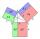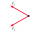# The Law of Cosines - math problems

#### Number of problems found: 42

• Four sides of trapezoidIn the trapezoid ABCD is |AB| = 73.6 mm; |BC| = 57 mm; |CD| = 60 mm; |AD| = 58.6 mm. Calculate the size of its interior angles.
• Medians of isosceles triangleThe isosceles triangle has a base ABC |AB| = 16 cm and 10 cm long arm. What is the length of medians?
• Triangle ABCTriangle ABC has side lengths m-1, m-2, m-3. What has to be m to be triangle a) rectangular b) acute-angled?
• Diagonals in diamondIn the rhombus is given a = 160 cm, alpha = 60 degrees. Calculate the length of the diagonals.
• Scalene triangleSolve the triangle: A = 50°, b = 13, c = 6
• Find the areaFind the area of the triangle with the given measurements. Round the solution to the nearest hundredth if necessary. A = 50°, b = 30 ft, c = 14 ft
• DiagonalsCalculate the length of the rhombus's diagonals if its side is long 5 and one of its internal angles is 80°.
• Greatest angleCalculate the greatest triangle angle with sides 197, 208, 299.
• Two boatsTwo boats are located from a height of 150m above the surface of the lake at depth angles of 57° and 39°. Find the distance of both boats if the sighting device and both ships are in a plane perpendicular to the surface of the lake.
• Side cIn △ABC a=2, b=4 and ∠C=100°. Calculate length of the side c.
• Triangle and its heightsCalculate the length of the sides of the triangle ABC, if va=5 cm, vb=7 cm and side b is 5 cm shorter than side a.
• LawsFrom which law follows directly the validity of Pythagoras' theorem in the right triangle? ...
• Heron backlawCalculate missing side in a triangle with sides 25 and 13 and area 152.
• Triangle SASCalculate the triangle area and perimeter, if the two sides are 51 cm and 110 cm long and angle them clamped is 130 °.
• Mass pointTwo equal forces of 30 Newtons act on a mass point. Find the magnitude of the resultant force if these forces form an angle of 42°.
• Space vectors 3DThe vectors u = (1; 3; -4), v = (0; 1; 1) are given. Find the size of these vectors, calculate the angle of the vectors, the distance between the vectors.
• TrianglePlane coordinates of vertices: K[11, -10] L[10, 12] M[1, 3] give Triangle KLM. Calculate its area and its interior angles.
• Vector sumThe magnitude of the vector u is 12 and the magnitude of the vector v is 8. Angle between vectors is 61°. What is the magnitude of the vector u + v?
• Three vectorsThe three forces whose amplitudes are in ratio 9:10:17 act in the plane at one point to balance. Determine the angles of each two forces.What is the volume of a quadrilateral oblique prism with base edges of length a = 1m, b = 1.1m, c = 1.2m, d = 0.7m, if a side edge of length h = 3.9m has a deviation from the base of 20° 35' and the edges a, b form an angle of 50.5°.